# 生成对抗网络简介 (GAN Introduction)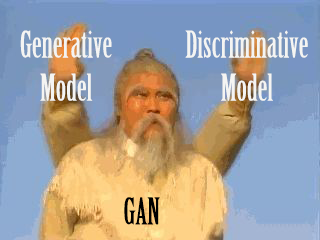## 网络框架

GAN 由两部分构成，一个生成器 (Generator) 和一个判别器 (Discriminator)。对于生成器，我们需要学习关于数据 $\boldsymbol{x}$ 的一个分布 $p_g$，首先定义一个输入数据的先验分布 $p_{\boldsymbol{z}} \left(\boldsymbol{z}\right)$，其次定义一个映射 $G \left(\boldsymbol{z}; \theta_g\right): \boldsymbol{z} \to \boldsymbol{x}$。对于判别器，我们则需要定义一个映射 $D \left(\boldsymbol{x}; \theta_d\right)$ 用于表示数据 $\boldsymbol{x}$ 是来自于真实数据，还是来自于 $p_g$。GAN 的网络框架如下图所示 2## 模型训练

Goodfellow 在文献中给出了一个重要的公式用于求解最优的生成器

$$\min_{G} \max_{D} V\left(D, G\right) = \mathbb{E}_{\boldsymbol{x} \sim p_{data}{\left(\boldsymbol{x}\right)}}{\left[\log D\left(\boldsymbol{x}\right)\right]} + \mathbb{E}_{\boldsymbol{z} \sim p_{\boldsymbol{z}}\left(\boldsymbol{z}\right)}{\left[\log \left(1 - D\left(G\left(\boldsymbol{z}\right)\right)\right)\right]}$$

$$\begin{equation} \begin{split} V \left(G, D\right) &= \mathbb{E}_{\boldsymbol{x} \sim p_{data}{\left(\boldsymbol{x}\right)}}{\left[\log D\left(\boldsymbol{x}\right)\right]} + \mathbb{E}_{\boldsymbol{z} \sim p_{\boldsymbol{z}}\left(\boldsymbol{z}\right)}{\left[\log \left(1 - D\left(G\left(\boldsymbol{z}\right)\right)\right)\right]} \\ &= \int_{\boldsymbol{x}}{p_{data}\left(\boldsymbol{x}\right) \log D\left(\boldsymbol{x}\right) dx} + \int_{\boldsymbol{z}}{p_{\boldsymbol{z}} \left(\boldsymbol{z}\right) \log \left(1 - D\left(g\left(\boldsymbol{z}\right)\right)\right) dz} \\ &= \int_{\boldsymbol{x}}{p_{data}\left(\boldsymbol{x}\right) \log D\left(\boldsymbol{x}\right) + p_g\left(\boldsymbol{x}\right) \log \left(1 - D\left(\boldsymbol{x}\right)\right) dx} \end{split} \end{equation}$$

$$D_{G}^{*} = \dfrac{p_{data} \left(\boldsymbol{x}\right)}{p_{data} \left(\boldsymbol{x}\right) + p_g \left(\boldsymbol{x}\right)}$$

$$\begin{equation} \begin{split} &C\left(G\right) \\ =& \max_{D} V \left(G, D\right) = V \left(G, D^*\right) \\ =& \mathbb{E}_{\boldsymbol{x} \sim p_{data}{\left(\boldsymbol{x}\right)}}{\left[\log D_{G}^{*}\left(\boldsymbol{x}\right)\right]} + \mathbb{E}_{\boldsymbol{z} \sim p_{\boldsymbol{z}}\left(\boldsymbol{z}\right)}{\left[\log \left(1 - D_{G}^{*}\left(G\left(\boldsymbol{z}\right)\right)\right)\right]} \\ =& \mathbb{E}_{\boldsymbol{x} \sim p_{data}{\left(\boldsymbol{x}\right)}}{\left[\log D_{G}^{*}\left(\boldsymbol{x}\right)\right]} + \mathbb{E}_{\boldsymbol{x} \sim p_g\left(\boldsymbol{x}\right)}{\left[\log \left(1 - D_{G}^{*}\left(\boldsymbol{x}\right)\right)\right]} \\ =& \mathbb{E}_{\boldsymbol{x} \sim p_{data}{\left(\boldsymbol{x}\right)}}{\left[\log \dfrac{p_{data} \left(\boldsymbol{x}\right)}{p_{data} \left(\boldsymbol{x}\right) + p_g \left(\boldsymbol{x}\right)} \right]} + \mathbb{E}_{\boldsymbol{x} \sim p_g\left(\boldsymbol{x}\right)}{\left[\log \dfrac{p_g \left(\boldsymbol{x}\right)}{p_{data} \left(\boldsymbol{x}\right) + p_g \left(\boldsymbol{x}\right)}\right]} \\ =& \int_{x}{p_{data} \left(\boldsymbol{x}\right) \log \dfrac{\dfrac{1}{2} p_{data} \left(\boldsymbol{x}\right)}{\dfrac{p_{data} \left(\boldsymbol{x}\right) + p_g \left(\boldsymbol{x}\right)}{2}} dx} + \int_{x}{p_g \left(\boldsymbol{x}\right) \log \dfrac{\dfrac{1}{2} p_g \left(\boldsymbol{x}\right)}{\dfrac{p_{data} \left(\boldsymbol{x}\right) + p_g \left(\boldsymbol{x}\right)}{2}} dx} \\ =& \int_{x}{p_{data} \left(\boldsymbol{x}\right) \log \dfrac{p_{data} \left(\boldsymbol{x}\right)}{\dfrac{p_{data} \left(\boldsymbol{x}\right) + p_g \left(\boldsymbol{x}\right)}{2}} dx} + \int_{x}{p_g \left(\boldsymbol{x}\right) \log \dfrac{p_g \left(\boldsymbol{x}\right)}{\dfrac{p_{data} \left(\boldsymbol{x}\right) + p_g \left(\boldsymbol{x}\right)}{2}} dx} + 2 \log \dfrac{1}{2} \\ =& KL \left(p_{data} \left(\boldsymbol{x}\right) \Vert \dfrac{p_{data} \left(\boldsymbol{x}\right) + p_g \left(\boldsymbol{x}\right)}{2}\right) + KL \left(p_g \left(\boldsymbol{x}\right) \Vert \dfrac{p_{data} \left(\boldsymbol{x}\right) + p_g \left(\boldsymbol{x}\right)}{2}\right) - 2 \log 2 \\ =& 2 JS \left(p_{data} \left(\boldsymbol{x}\right) \Vert p_g \left(\boldsymbol{x}\right) \right) - 2 \log 2 \end{split} \end{equation}$$

\begin{algorithm}
\caption{Minibatch SGD for GAN 算法}
\begin{algorithmic}
\REQUIRE $iter, k, m$
\ENSURE $\theta_d, \theta_g$
\FOR{$i = 1, 2, ..., iter$}
\FOR{$j = 1, 2, ..., k$}
\STATE Sample minibatch of $m$ noise samples $\{z^{\left(1\right)}, ..., z^{\left(m\right)}\}$ from $p_g \left(\boldsymbol{z}\right)$
\STATE Sample minibatch of $m$ examples $\{x^{\left(1\right)}, ..., x^{\left(m\right)}\}$ from $p_{data} \left(\boldsymbol{z}\right)$
\STATE $\theta_d \gets \theta_d \textcolor{red}{+} \nabla_{\theta_d} \dfrac{1}{m} \sum_{i=1}^{m}{\left[\log D \left(x^{\left(i\right)}\right) + \log \left(1 - D \left(G \left(z^{\left(i\right)}\right)\right)\right)\right]}$
\ENDFOR
\STATE Sample minibatch of $m$ noise samples $\{z^{\left(1\right)}, ..., z^{\left(m\right)}\}$ from $p_g \left(\boldsymbol{z}\right)$
\STATE $\theta_g \gets \theta_g \textcolor{red}{-} \nabla_{\theta_g} \dfrac{1}{m} \sum_{i=1}^{m}{\log \left(1 - D \left(G \left(z^{\left(i\right)}\right)\right)\right)}$
\ENDFOR
\end{algorithmic}
\end{algorithm}


## 存在的问题

### JS 散度问题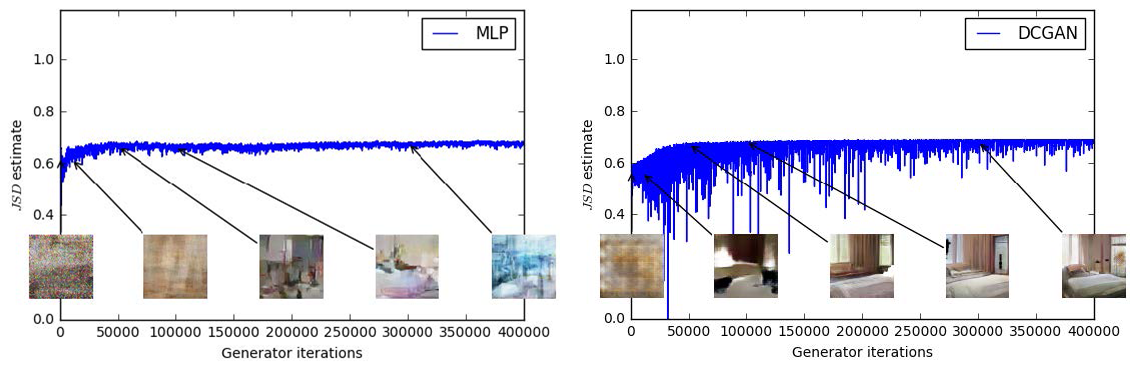$$\begin{equation} \begin{split} L \left(D, \theta_g\right) &= \mathbb{E}_{\boldsymbol{x} \sim p_{data}{\left(\boldsymbol{x}\right)}}{\left[\log D_{G}^{*}\left(\boldsymbol{x}\right)\right]} + \mathbb{E}_{\boldsymbol{x} \sim p_g\left(\boldsymbol{x}\right)}{\left[\log \left(1 - D_{G}^{*}\left(\boldsymbol{x}\right)\right)\right]} \\ &= 2 JS \left(p_{data} \left(\boldsymbol{x}\right) \Vert p_g \left(\boldsymbol{x}\right) \right) - 2 \log 2 \end{split} \end{equation}$$

### 多样性问题 Mode Collapse

Mode Collapse 问题是指生成器更多的是生成了大量相同模式的数据，导致的结果就是生成的数据缺乏多样性，如下图所示 7: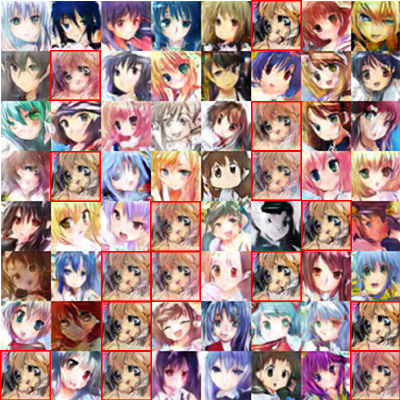• 基于正则化的优化 8
• 基于 Minibatch 的优化 9
• 基于 Unrolled Optimization 的优化 10
• 基于集成算法的优化 11

## MNIST 示例

• 生成器

def build_generator(self):
model = Sequential()

input_shape=self._noise_shape))

print('Generator Summary: ')
model.summary()

noise = Input(shape=self._noise_shape)
image = model(noise)

return Model(noise, image)


• 判别器

def build_discriminator(self):
model = Sequential()

print('Discriminator Summary: ')
model.summary()

image = Input(shape=self._input_shape)
label = model(image)

return Model(image, label)


• 对抗网络

class ImageBasicGAN():
def __init__(self, width, height, channels,
noise_dim=100, hidden_dim=1024):
'''

Args:
width: 图像宽度
height: 图像高度
channels: 图像颜色通道数
a_optimizer: 对抗网络优化器
g_optimizer: 生成器优化器
d_optimizer: 判别器优化器
noise_dim: 噪音数据维度
hidden_dim: 隐含层最大维度
'''

# 省略一大坨代码

# 构建和编译判别器
self._discriminator = self.build_discriminator()
self._discriminator.compile(loss='binary_crossentropy',
optimizer=d_optimizer,
metrics=['accuracy'])

# 构建和编译生成器
self._generator = self.build_generator()
self._generator.compile(loss='binary_crossentropy',
optimizer=g_optimizer)

# 生成器利用噪声数据作为输入
noise = Input(shape=self._noise_shape)
generated_image = self._generator(noise)

# 当训练整个对抗网络时，仅训练生成器
self._discriminator.trainable = False

# 判别器将生成的图像作为输入
label = self._discriminator(generated_image)

# 构建和编译整个对抗网络
optimizer=a_optimizer)


• 训练过程

def train(self, x_train, output_dir, iters,
batch_size=32, k=1, save_interval=200):
''' 训练模型

Args:
x_train: 训练数据
output_dir: 相关输出路径
iters: 迭代次数
batch_size: 批大小
k: K
save_interval: 结果保存间隔
'''

# 省略一大坨代码

for iter in range(iters):
# 训练判别器
for _ in range(k):
train_indices = np.random.randint(0, x_train.shape,
batch_size)
train_images = x_train[train_indices]

noises = np.random.normal(0, 1, (batch_size, self._noise_dim))
generated_images = self._generator.predict(noises)

self._discriminator.train_on_batch(train_images,
np.ones((batch_size, 1)))
self._discriminator.train_on_batch(generated_images,
np.zeros((batch_size, 1)))

# 训练生成器
noises = np.random.normal(0, 1, (batch_size, self._noise_dim))
labels = np.ones(batch_size)

# 再省略一大坨代码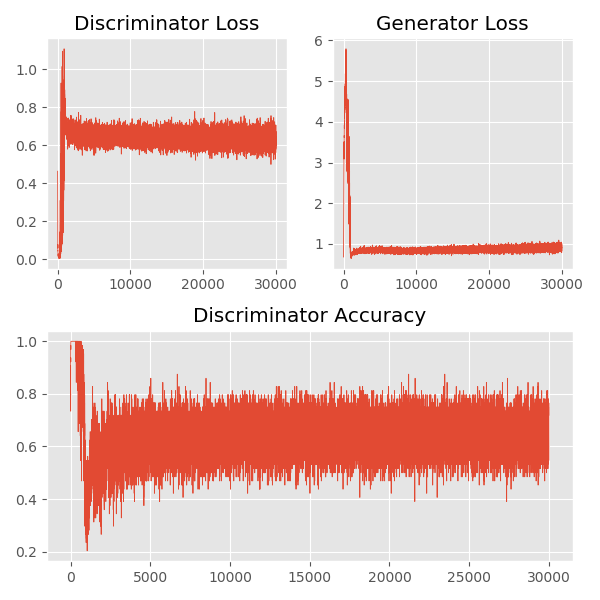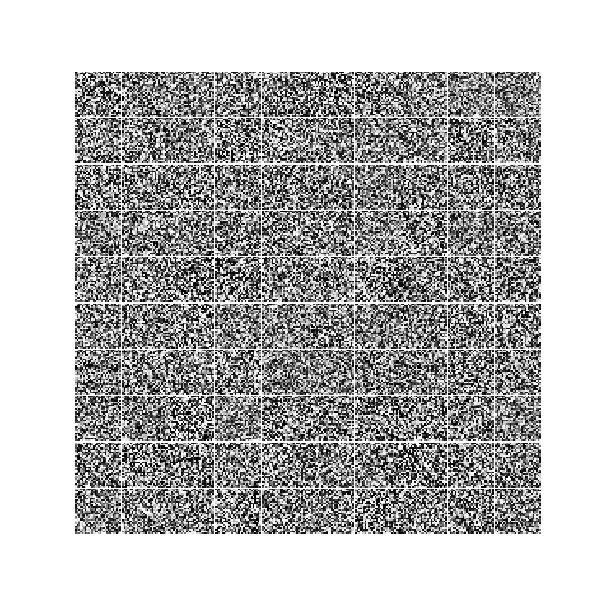# Deep Convolutional GAN

DCGAN (Deep Convolutional GAN) 是由 Radford 6 等人提出的一种对原始 GAN 的变种，其基本的思想就是将原始 GAN 中的全链接层用卷积神经网络代替。在文中，Radford 等人给出构建一个稳定的 DCGAN 的建议，如下：

• 在网络中不使用 pooling 层，而是使用多步长的卷积层 (判别器) 和多步长的反卷积层 (生成器)。
• 在生成器和判别器中均使用批标准化。
• 对于深层的框架，去掉全链接层。
• 在生成器中使用 ReLU 激活函数，最后一层使用 Tanh 激活函数。
• 在判别器中使用 LeakyReLU 激活函数。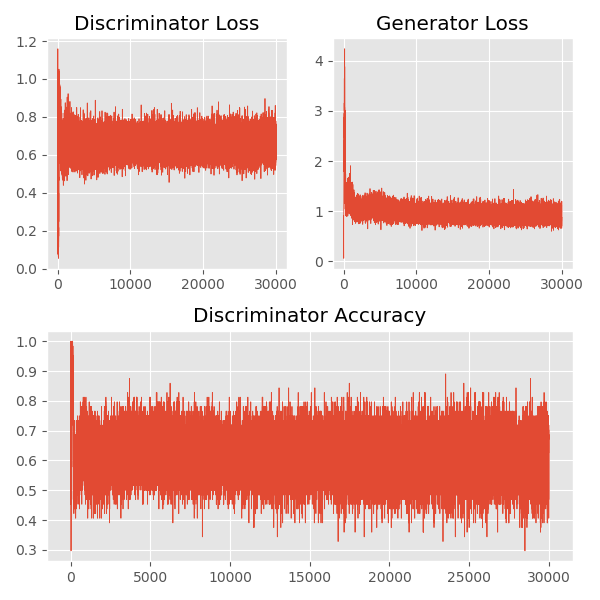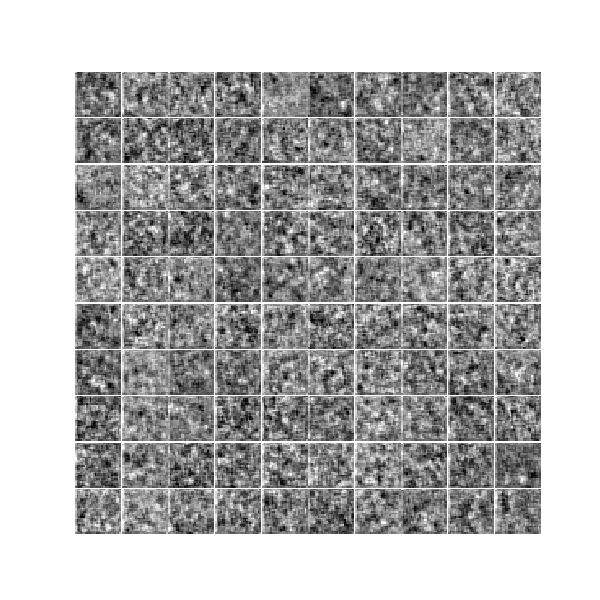1. Goodfellow, Ian, et al. “Generative adversarial nets.” Advances in neural information processing systems. 2014. ↩︎

2. Goodfellow, Ian. “NIPS 2016 tutorial: Generative adversarial networks.” arXiv preprint arXiv:1701.00160 (2016). ↩︎ ↩︎

3. https://en.wikipedia.org/wiki/Kullback–Leibler_divergence ↩︎

4. https://en.wikipedia.org/wiki/Jensen–Shannon_divergence ↩︎

5. Arjovsky, Martin, Soumith Chintala, and Léon Bottou. “Wasserstein gan.” arXiv preprint arXiv:1701.07875 (2017). ↩︎

6. Radford, Alec, Luke Metz, and Soumith Chintala. “Unsupervised representation learning with deep convolutional generative adversarial networks.” arXiv preprint arXiv:1511.06434 (2015). ↩︎ ↩︎

7. Che, Tong, et al. “Mode regularized generative adversarial networks.” arXiv preprint arXiv:1612.02136 (2016). ↩︎

8. Salimans, Tim, et al. “Improved techniques for training gans.” Advances in Neural Information Processing Systems. 2016. ↩︎

9. Metz, Luke, et al. “Unrolled generative adversarial networks.” arXiv preprint arXiv:1611.02163 (2016). ↩︎

10. Tolstikhin, Ilya O., et al. “Adagan: Boosting generative models.” Advances in Neural Information Processing Systems. 2017. ↩︎